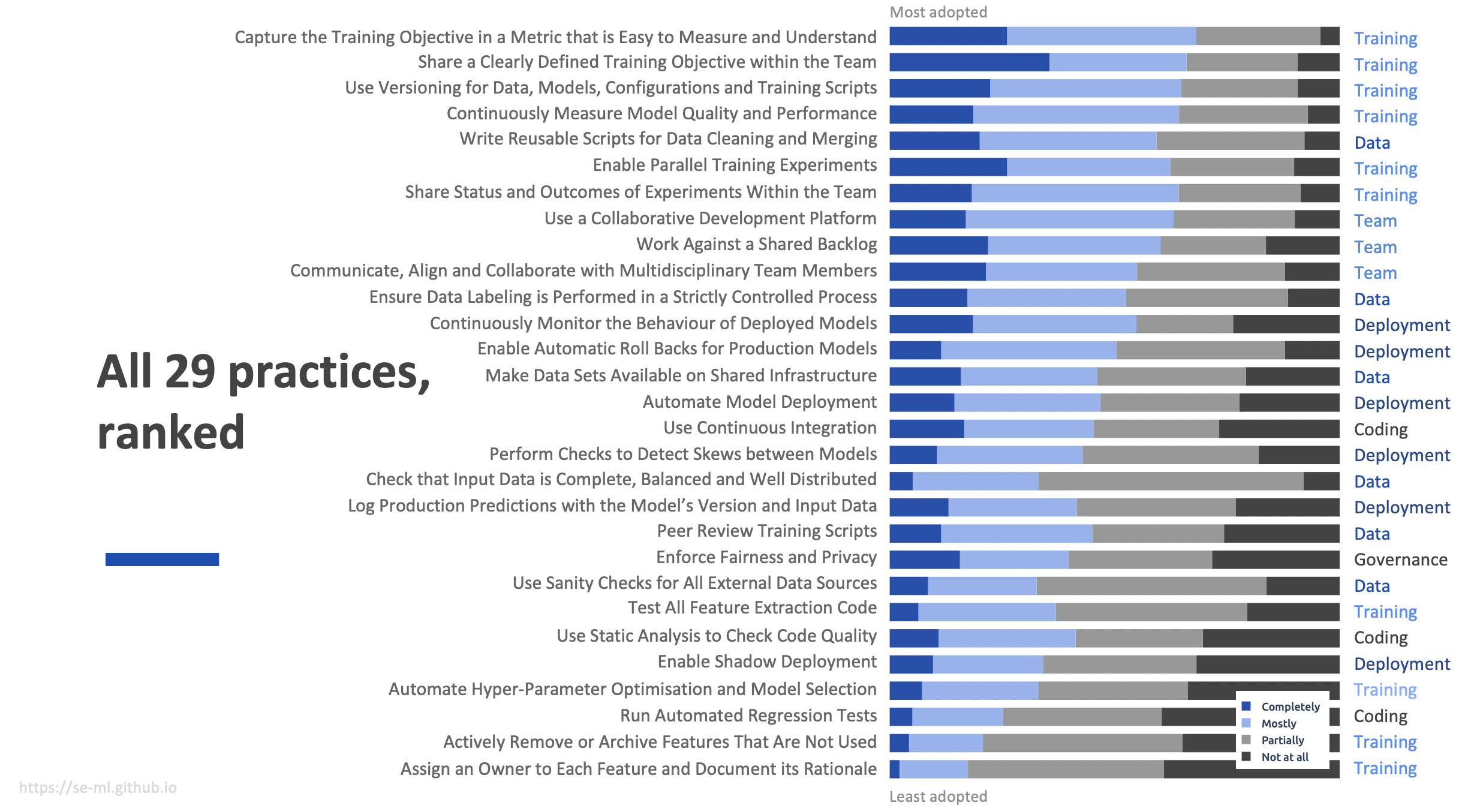Using the results from our survey, we ranked the practices based on three level of difficulty: basic, medium and advanced.

The ranking algorithm computes, for each practice, the percentage of “Completely” answers (corresponding to at least high adoption of a practice), the percentage of “Completely + Mostly” answers (corresponding to at least medium adoption of a practice) and the percentage of “Completely + Mostly + Partially” answers (corresponding to at least low adoption of a practice).

The final rank is the average of: the practice rank on at least high, its rank on at least medium, and its rank on at least low adoption. By accumulating the answers in this manner, we expect to cancel out the noise stemming from fuzzy boundaries between subsequent answer types or the relative unachievability of complete adoption of a particular practice.

We split the list of ranked practice in 3 percentiles corresponding to “basic” (first group with high adoption), “medium” (second group) and “advanced” (third group with low adoption).

An overview of the percentage of answers for each practice is illustrated below.